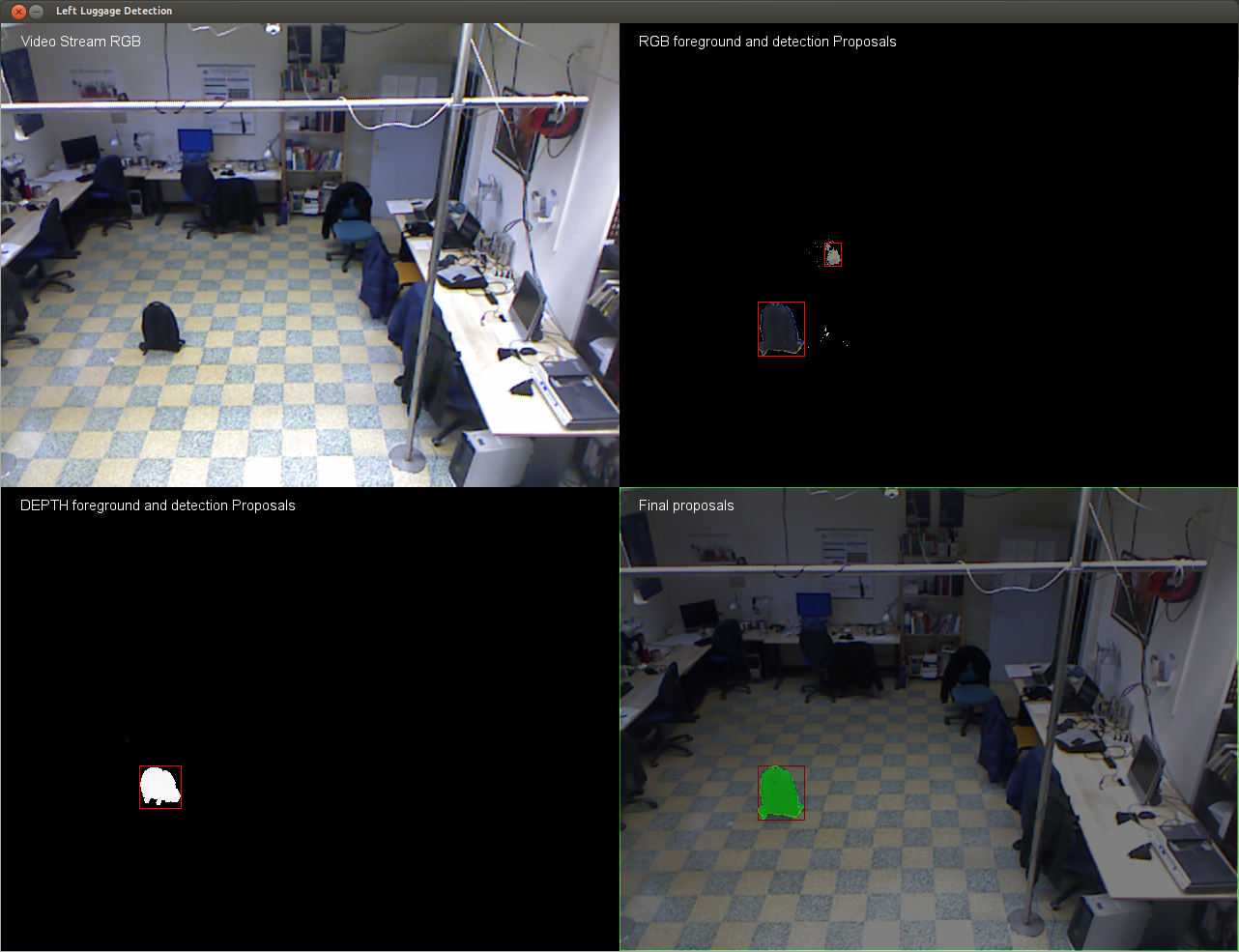# Combination of proposals¶

Given the two sets of bounding box obtained through the processing of depth and intensity video streams, we compute the following pairwise overlap ratios:

$$r = \frac{area \left(B_{curr} \cap B_{acc} \right)}{area \left(B_{curr} \cup B_{acc} \right)}$$A possible luggage, obtained through the formula above, is no longer detected because of two possible reasons:

• a left item is removed from the scene
• the item detected is standing still for a long amount of time. After this time the item became part of the depth and rgb background. When the item became part of the background model we can’t detect its presence doing $$current\_frame - bg\_model$$ so we need a way to retain the information previously discovered. If a Bounding box is present at the frame t-1 but not in the frame t, we check if pixels in the area, defined by his bounding box, are still the same (i.e. the luggage is still there): this check is performed by using the normalized correlation between the pixel in the t-1 and t frames. If the similarity is above a certain threshold (i.e. 0.9) we keep drawing the old bounding box.